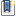File : Details

# Newton basins of attraction

Draws the basins of attraction of known solutions for the Newton iteration
Details
Version
1.0
Author
Jérôme LABATUT
Maintainer
Jérôme LABATUT
Categories
Supported Scilab Version
Creation Date
May 27, 2016Description
```            Draws the basins of attraction for the Newton iteration of known solutions to
the following equations :

z^3 - z = 0
z^3 = 1
z^4 = 1
z^5 = 1
z^6 = 1

cos(z) = 1/2
sin(z) = 1/2
tan(z) = 1 (buggy : divide by zero)

ch(z) = 2
sh(z) = 2
th(z) = 1/2 (buggy for the same reason)

Button "Agrandir" allows user to zoom on the Newton basins.

Button "Restaurer" draws back with the default size settings.            ```Files (2)Source code archive
```
```Screenshot
```
```News (0)Comments (0)Leave a comment
Login withEmail notifications